# TExMaT Master Mathematics Teacher 8-12: Graphing Derivatives Chapter Exam

Exam Instructions:

Choose your answers to the questions and click 'Next' to see the next set of questions. You can skip questions if you would like and come back to them later with the yellow "Go To First Skipped Question" button. When you have completed the practice exam, a green submit button will appear. Click it to see your results. Good luck!

### Page 1

#### Question 1 1. What is the equation of the line tangent to f(x) at the point x=0?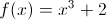#### Question 2 2. Between x=1 and x=3, the following function _____.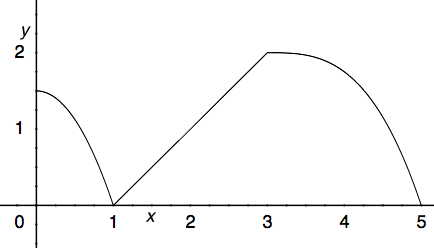#### Question 4 4. Consider the function f(x) below. At x = 2 is this function concave up, concave down, or an inflection point?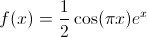#### Question 5 5. What is the slope of the tangent to r(s) at s = 0?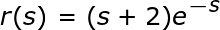### Page 2

#### Question 6 6. The graph shown is an example of a function that: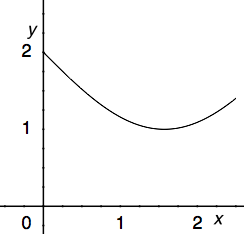#### Question 7 7. What is the global minimum for the function y=f(x) below between x=0 and x=2?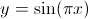#### Question 8 8. What is the equation of the line normal to f(x) at the point x=1?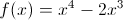#### Question 10 10. Which of the following BEST describes point A?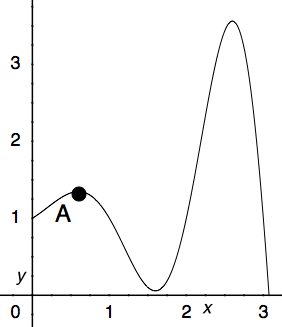### Page 3

#### Question 11 11. Which could be the graph of the derivative of the function below?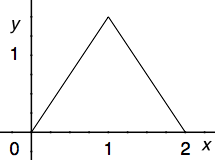#### Question 12 12. What is the global minimum for the function y=f(x) below between x=0 and x=2?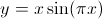#### Question 13 13. The derivative of the following graph is _____ at point A.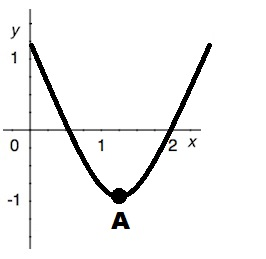#### Question 14 14. What is the slope of the line normal to f(x) at x=1?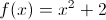### Page 4

#### Question 17 17. The graph shown is an example of a function that: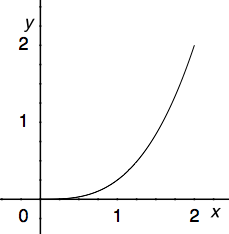#### Question 18 18. Consider the function h(x) below. At x = 1 is this function concave up, concave down, or an inflection point?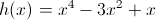#### Question 19 19. Which of the following BEST describes point B?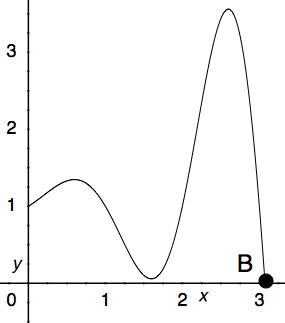#### Question 20 20. What is the slope of a line normal to f(x)?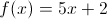### Page 5

#### Question 21 21. Where is the global maximum for the function y=f(x) below between x=0 and x=2?#### Question 22 22. The graph shown is an example of a function that: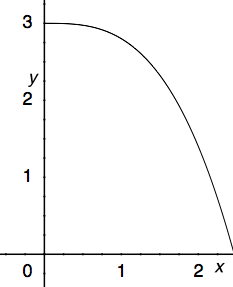#### Question 23 23. Which of the following BEST describes point C?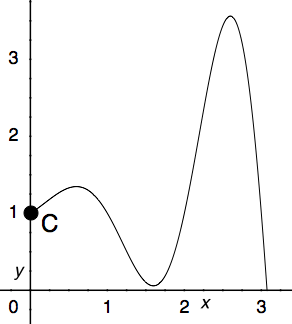#### Question 24 24. Which of the following is a local minimum?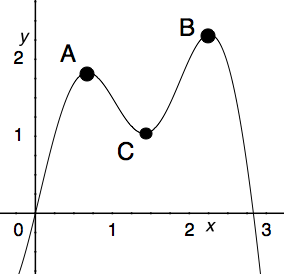#### Question 25 25. What is value of y at the finite local maximum for the function y=f(x) below?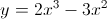### Page 6

#### Question 26 26. The derivative of the following graph is _____ at point D.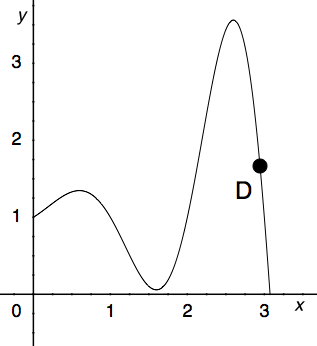#### Question 28 28. Consider the function f(x) below. At x = 1 is this function concave up, concave down, or an inflection point?#### Question 30 30. Over the region shown, point A is known as _____.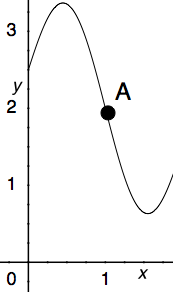#### TExMaT Master Mathematics Teacher 8-12: Graphing Derivatives Chapter Exam Instructions

Choose your answers to the questions and click 'Next' to see the next set of questions. You can skip questions if you would like and come back to them later with the yellow "Go To First Skipped Question" button. When you have completed the practice exam, a green submit button will appear. Click it to see your results. Good luck!

Support